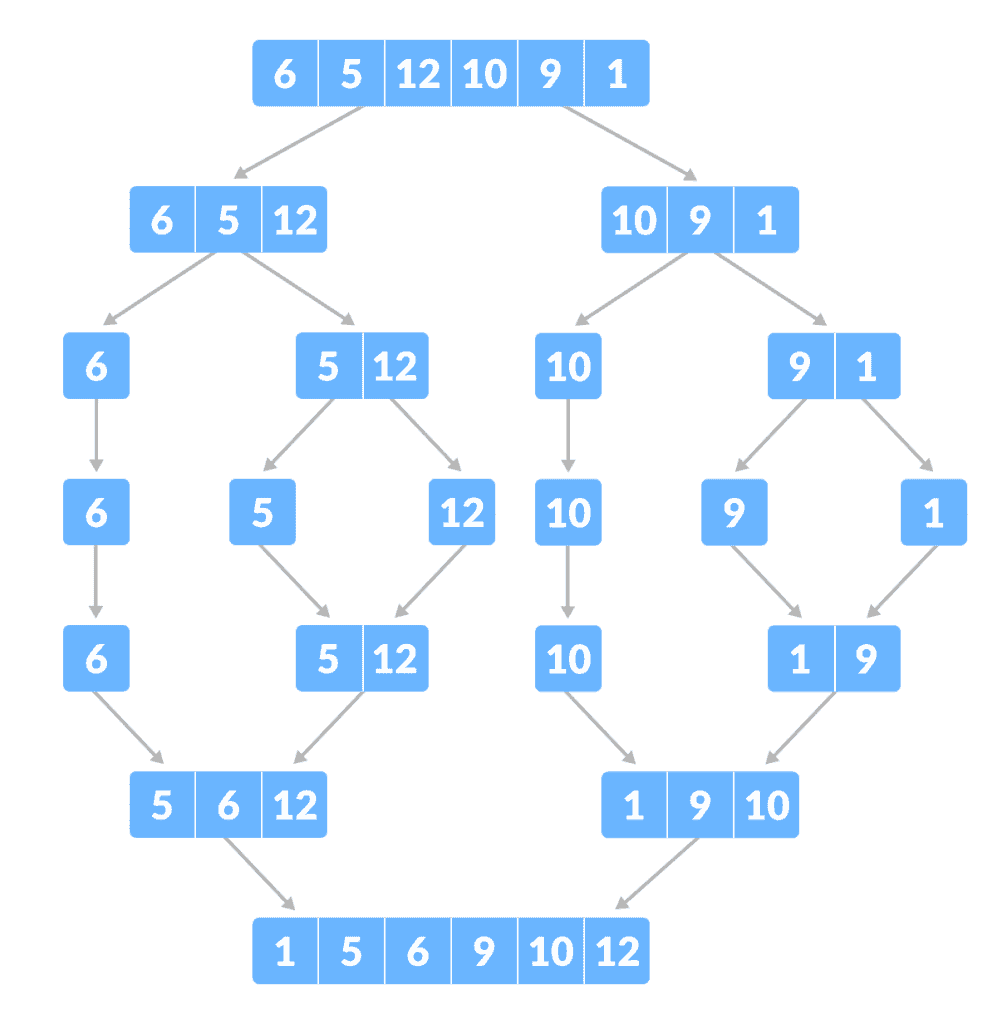# Merge Sort Algorithm in C Programming Language

Merge Sort Algorithm in C language is a quick sort algorithm for sorting. It is one of the most popular sorting algorithms in computer programming. It is also known as Divide and Conquer algorithm.

This is the most efficient sorting algorithms in c or java language. Merge sort repeatedly breaks down the given list into several sub-lists until each sub-list consists of a single element and merging those sub lists results into a sorted list.## C Language – Merge Sort Algorithm:

MergeSort(A, p, r):
if p > r
return
q = (p+r)/2
mergeSort(A, p, q)
mergeSort(A, q+1, r)
merge(A, p, q, r)

### Merge Sort Algorithm Program Code:

```#include<stdlib.h>
#include<stdio.h>
// Merge Function
void merge(int arr[], int l, int m, int r)
{
int i, j, k;
int n1 = m - l + 1;
int n2 = r - m;
int L[n1], R[n2];
for (i = 0; i < n1; i++)
L[i] = arr[l + i];
for (j = 0; j < n2; j++)
R[j] = arr[m + 1+ j];
i = 0;
j = 0;
k = l;
while (i < n1 && j < n2)
{
if (L[i] <= R[j])
{
arr[k] = L[i];
i++;
}
else
{
arr[k] = R[j];
j++;
}
k++;
}
while (i < n1)
{
arr[k] = L[i];
i++;
k++;
}
while (j < n2)
{
arr[k] = R[j];
j++;
k++;
}
}```

You can try this code in Borland C++ IDE, as it supports the C language also.

Also Read: Banker’s Algorithm in Operating System

#### Merge Sort Algorithm in C Example:

```#include <stdio.h>

#define max 10

int a = { 10, 14, 19, 26, 27, 31, 33, 35, 42, 44, 0 };
int b;

void merging(int low, int mid, int high) {
int l1, l2, i;

for(l1 = low, l2 = mid + 1, i = low; l1 <= mid && l2 <= high; i++) {
if(a[l1] <= a[l2])
b[i] = a[l1++];
else
b[i] = a[l2++];
}

while(l1 <= mid)
b[i++] = a[l1++];

while(l2 <= high)
b[i++] = a[l2++];

for(i = low; i <= high; i++)
a[i] = b[i];
}

void sort(int low, int high) {
int mid;

if(low < high) {
mid = (low + high) / 2;
sort(low, mid);
sort(mid+1, high);
merging(low, mid, high);
} else {
return;
}
}

int main() {
int i;

printf("List before sorting\n");

for(i = 0; i <= max; i++)
printf("%d ", a[i]);

sort(0, max);

printf("\nList after sorting\n");

for(i = 0; i <= max; i++)
printf("%d ", a[i]);
}```

Output:

```List before sorting
10 14 19 26 27 31 33 35 42 44 0
List after sorting
0 10 14 19 26 27 31 33 35 42 44```

Merge Sort Algorithm works on the Divide and Conquer principle. If you still having difficulty understanding, then you can watch the below video tutorial to understand Merge Sort Algorithm clearly.

### Conclusion:

You can check the source code of this program in this article itself. Feel free to contact us if you have any questions regarding merge sort in c language or anything else.